# `<ST_LightRigDirection>` (Light Rig Direction)

Represents the direction from which the light rig is positioned relative to the scene. The light rig, itself, can be made up of multiple lights in any orientation around a given shape. This simple type defines the orientation of the light rig as a whole, and not the individual lights within the rig. This means that because the direction of the light rig is left, that does not guarantee the light will be coming from the left side of the shape, but rather the orientation of the rig as a whole is rotated to the left.

example: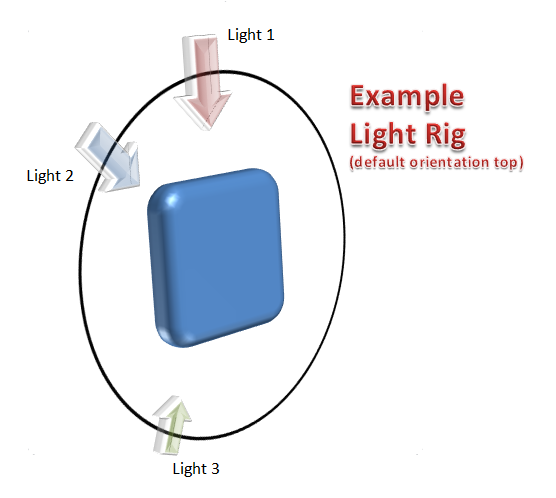In this example we see that the light rig defines three lights (all in a single plane as represented by the black circular line). The lights defined in this representation can all have different intensities, which means, for this example, Light 3 and Light 2 look to have a more intense effect (or could even be a different color) than Light 1. One can image rotating this rig to the so that Light 1 is to the right of the shape when the light rig direction is defined to be `@right`. ]

The following properties were used to define the shape used in the image examples below:

• Rounded rectangle shape

• Three Point light rig type

• Circle bevel type

• Plastic material type

• Camera type defined by the `orthographicFront` preset

• Bevel width and height each equal to `190500`

This simple type's contents are a restriction of the XML Schema token datatype.

The following are possible enumeration values for this type:

Enumeration Value

Description

`<b>` (Bottom)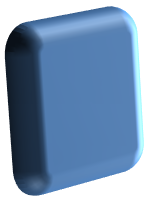`<bl>` (Bottom Left)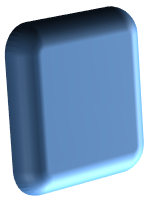`<br>` (Bottom Right)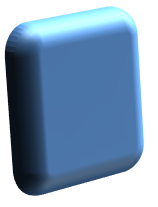`<l>` (Left)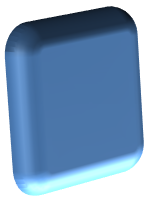`<r>` (Right)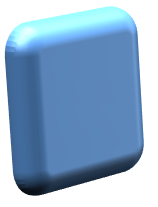`<t>` (Top)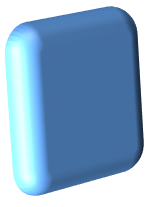`<tl>` (Top Left)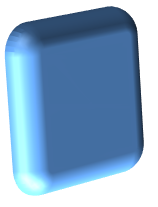`<tr>` (Top Right)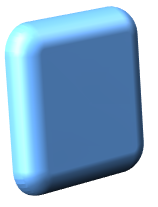Referenced By

`<lightRig@dir>`5.1.7.9)

The following XML Schema fragment defines the contents of this simple type:

``````<simpleType name="ST_LightRigDirection">
<restriction base="xsd:token">
<enumeration value="tl"/>
<enumeration value="t"/>
<enumeration value="tr"/>
<enumeration value="l"/>
<enumeration value="r"/>
<enumeration value="bl"/>
<enumeration value="b"/>
<enumeration value="br"/>
</restriction>
</simpleType>``````